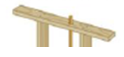Chapter 10, Problem 50P

Chapter
Section
Textbook Problem

In a period of 1.0 s, 5.0 × 1023 nitrogen molecules strike a wall of area 8.0 cm2. If the molecules move at 3.00 × 102 m/s and strike the wall head-on in a perfectly elastic collision, find the pressure exerted on the wall. (The mass of one N2 molecule is 4.68 × 10−26 kg.)To determine
The pressure exerted on the wall.

Explanation

Given Info: The time interval is 1 second. Mass of one nitrogen molecule is 4.68×1026kg . Speed of the molecules is 3.00×102m/s . Area (A) is 8.0cm2 . Number of molecules (N) fired is 5.0×1023 .

Formula to calculate the average force is,

F=N(m[vv']t)       (I)

• m is the mass of the bullet
• t  is the time interval
• v and v' are the velocities

Formula to calculate pressure is,

P=FA        (II)

From Equations (I) and (II),

P=(NA)(m[vv']t)

Substitute 5.0×1023 for N, 4.68×1026kg for m, 8.0cm2 for A,1 s for t, 3.00×102m/s for v and 3.00×102m/s for v' in the above expression to get F

Still sussing out bartleby?

Check out a sample textbook solution.

See a sample solution

The Solution to Your Study Problems

Bartleby provides explanations to thousands of textbook problems written by our experts, many with advanced degrees!

Get Started

Find more solutions based on key concepts